BioXing™                                                                                                 Solutions for Biotechnology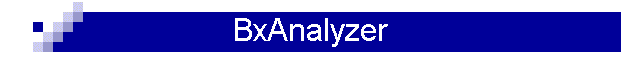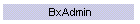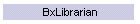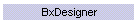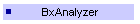# Analyzer

 The BxAnalyzertm provides basic analysis of  acquired raw data.This diagram illustrates that Raw Expression Data, Normalized Expression Data and Summarized Expression Data with their attributes and the parameters for Normalization and Summarization algorithms are stored in the BxArray databases. Normalization is the process where the values are scaled to allow comparisons with other experimental data sets.  For example, scaling can be done by using internal controls within the data set and adjusting the control values to a reference set of control values to obtain a scaling factor. Summarization is the process where values are grouped or averaged together to obtain a single representative value. A typical grouping is when there are duplicate measurements within the same data set. Also in the drawing are examples of Attributes that are saved for each value and Parameters for each algorithm. This implies that the set of results from applying algorithms with different data parameters can be saved or just the latest calculation is saved. Channel refers to measurements from a different wavelength.

Home ] Up ]